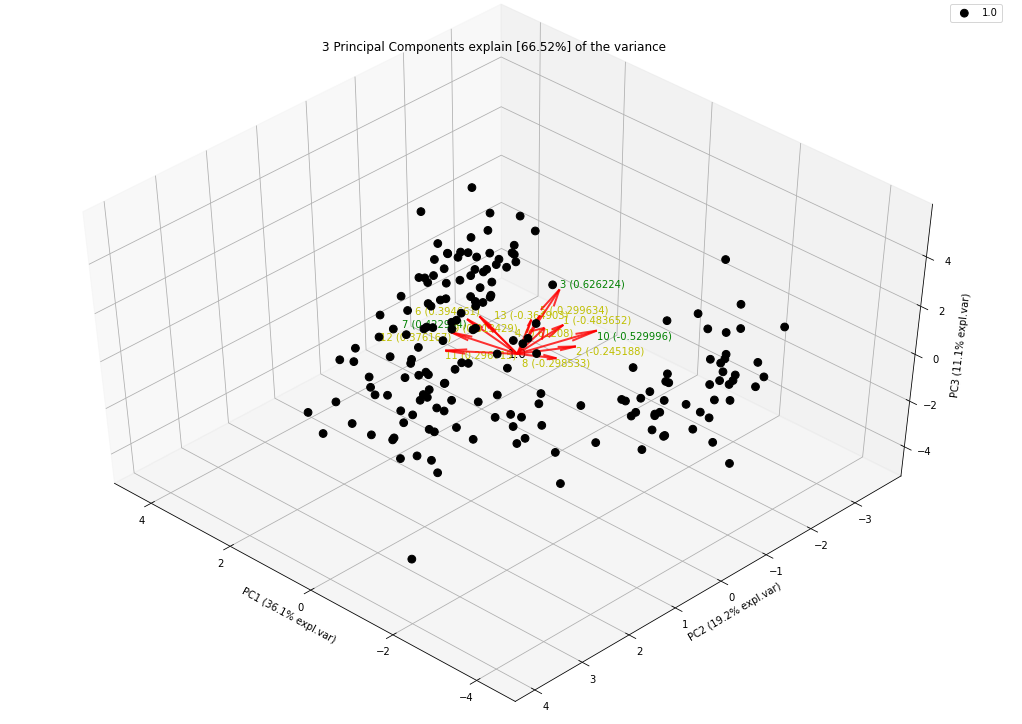Course Content

Principal Component AnalysisExplain Resulting Components

At the very beginning, we touched on the topic of interpreting the results obtained. First of all, it is worth mentioning that there is no universal method, there is only the possibility of expert evaluation, which depends entirely on how well you know the data you are working with. We have already mentioned the factor loading matrix. This matrix allows you to evaluate the contribution of each variable to the principal components. Formally, this means that each component is represented by a linear combination of initial variables Xn and coefficients wn:Thus, the loadings - are the coefficients wn of a linear combination of the initial variables from which the principal components are built. We have already visualized the factor loading matrix, but now you will get acquainted with a more convenient option. With the help of the `pca` library, you can easily create a visualization of loadings in 2D and 3D space:3D plot:We create the graphs obtained above using the following code:

If you want to specify labels for data classes, add an argument `row_labels` with y value for the function `fit_transform()`.